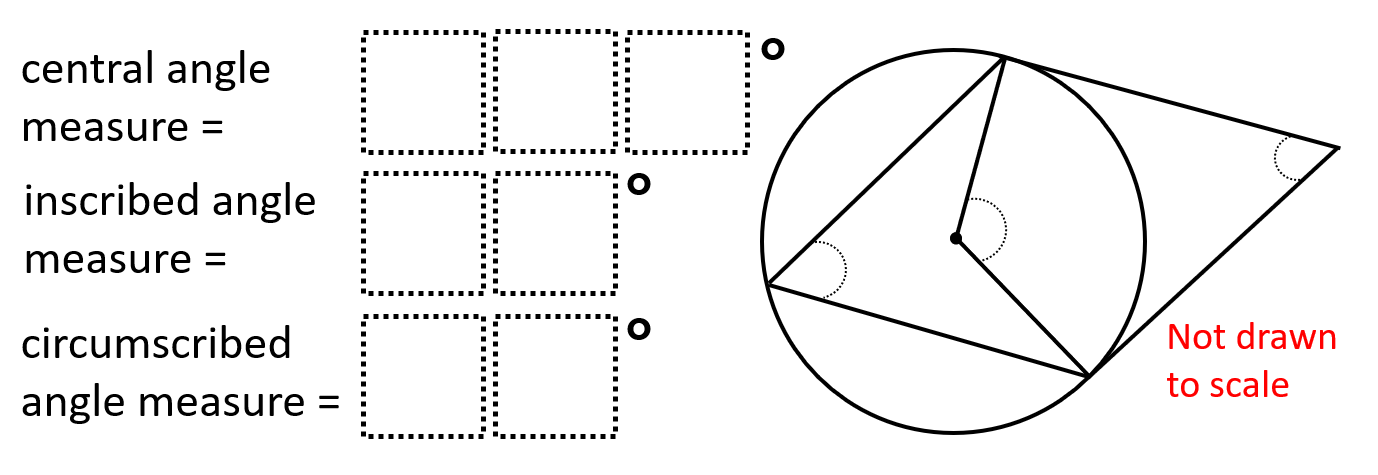# Central, Inscribed, & Circumscribed Angles 1

Directions: Using the digits 0 to 9 at most one time each, place a digit in each box two times: once where the central angle is greater than 130° and once where it is less than 130°. You may reuse all the digits each time.### Hint

How can we figure out which central angles are or are not worth checking?

Central angle: 104°
Inscribed angle: 52°
Circumscribed angle: 76°

Central angle: 106°
Inscribed angle: 53°
Circumscribed angle: 74°

Central angle: 108°
Inscribed angle: 54°
Circumscribed angle: 72°

Central angle: 138°
Inscribed angle: 69°
Circumscribed angle: 42°

Central angle: 156°
Inscribed angle: 78°
Circumscribed angle: 24°

Source: Robert Kaplinsky

## Similar Triangles 2

Directions: Using the digits 0 to 9 at most one time each, create two similar …